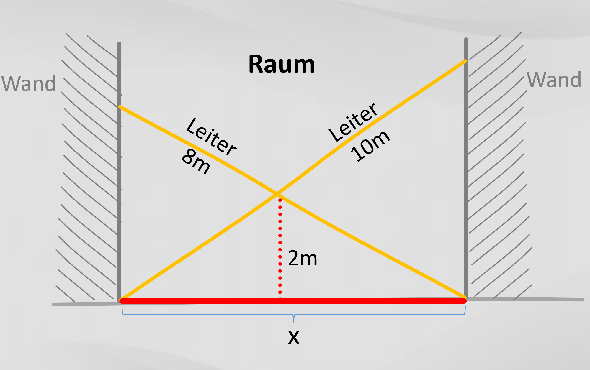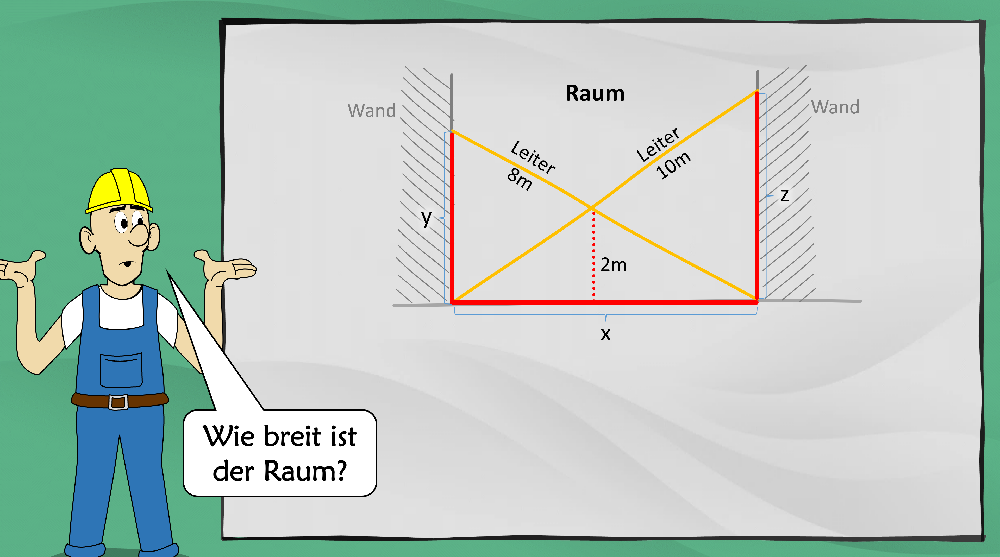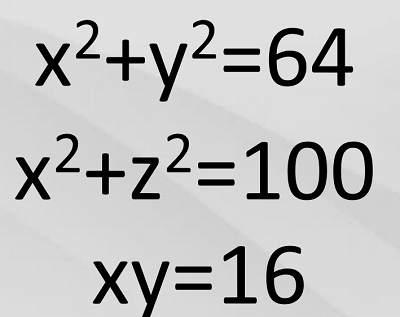In the context of post-quantum cryptography, the problem of crossed conductors plays a role. It is simple to explain, but difficult to solve.

Deutsche Version

More than 20 years ago I had a conversation with the cryptologist Prof. Hans Dobbertin, who has unfortunately passed away in the meantime. We came rather accidentally to a mathematical knobble, which I had discussed shortly before with some seminar participants to the re-entry after the lunch break (to overcome the “soup coma”).

It was about the problem of crossed ladders. This is a mathematical problem that apparently a middle school student can solve – with the ray theorem and the Pythagorean theorem. But what looks quite simple turns out to be almost unsolvable. Of the several dozen seminar participants to whom I presented the puzzle at the time, none was able to solve it. There were even mathematicians among the participants.

Hans Dobbertin, however, looked at the puzzle only once and said: “I know how to do it! Such questions play a role for certain crypto processes I’m dealing with right now.”

Unfortunately, we didn’t get around to elaborating. But I assumed that Dobbertin, probably the most important German cryptologist ever, had not told any nonsense. Some years later, I realized what he had in mind at that time.

### The problem of crossed ladders

The problem of crossed ladders is shown in the following diagram (the given numbers are of course only examples):Given are two ladders in a room, which cross each other. The lengths of the ladders and the height of the crossing point are known. The question is: How wide is the room?

### How do you solve the problem?

To answer the question, you can use the ray theorem and the Pythagorean theorem to set up three equations. According to my calculations, they look like this:The question now is how to solve this system of equations. One does not get far with the Gauss algorithm. Hans Dobbertin, however, thought that there was another method. He probably thought of so-called Gröbner bases, because these play a role in post-quantum cryptography (see below).

Unfortunately, I never got around to looking into Gröbner bases. Can a reader here provide an understandable explanation and tell how to use them to solve the problem?

In the English Wikipedia there is a separate entry on the problem of crossed ladders. Several possible solutions are discussed there. Can a reader use them to solve the version presented here?

Presumably, one can also use a mathematics software that solves systems of equations for this purpose. Wolfram Alpha would probably be a possibility. Does any reader have experience with it?

### Application in post-quantum cryptography

The question remains, what connection between the problem of crossed conductors and modern cryptography Hans Dobbertin saw at that time.

Here, too, I know the answer in the meantime: The above system of equations belongs to the multivariate systems of equations. These in turn are the basis of multivariate cryptography, which belongs to asymmetric cryptography.

Multivariate cryptography includes, for example, “oil and vinegar” signatures – named after variables known as “oil variables” and “vinegar variables.” Multivariate cryptography is counted as post-quantum cryptography. Thus, the digital signatures that can be realized with it cannot be forged even with a quantum computer.

Multivariate cryptography is based on the fact that multivariate systems of equations are easy to construct but difficult to solve. The best known solution method uses the aforementioned Gröbner bases. However, this method is so costly that for larger systems of equations it takes several billions of years, even with the best computer.

I have now given numerous talks in which I present multivariate cryptography (and other post-quantum methods) using cartoons. Here is a slide I use in doing so:

I always use the problem of crossed ladders as a starting point. Although I have already worked through at least a dozen literature sources on multivariate cryptography, I have never encountered the problem of crossed ladders in this context. Apparently, I am the only one who uses this illustrative puzzle for this purpose.

Does any reader know of other illustrative mathematical problems that can be solved using a multivariate system of equations?

If you want to add a comment, you need to add it to the German version here.